Publicité

# Introduction to code optimization by dipankar

26 Aug 2014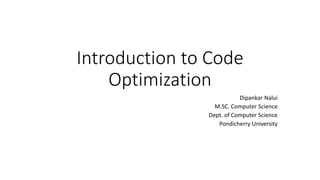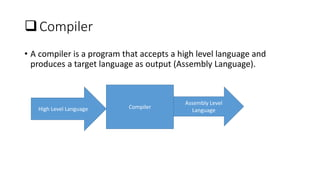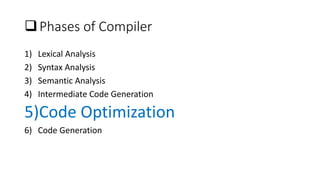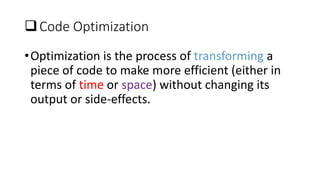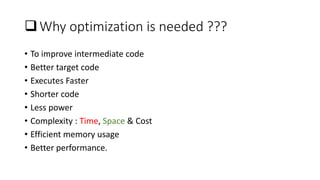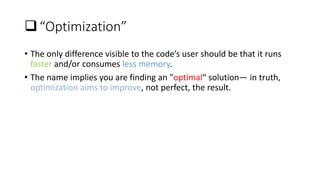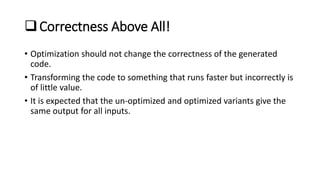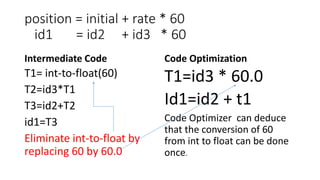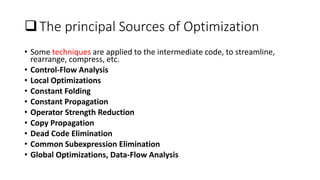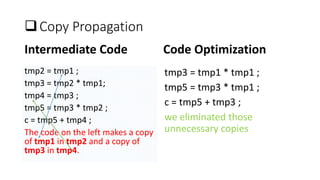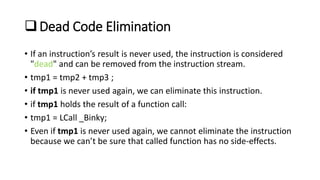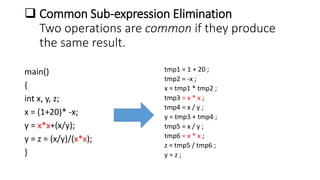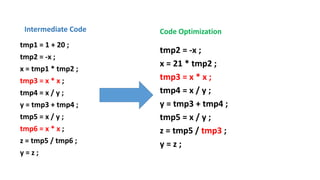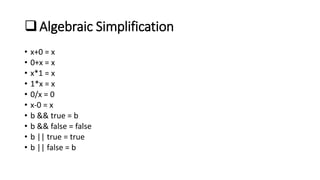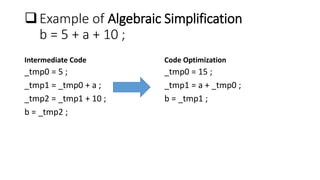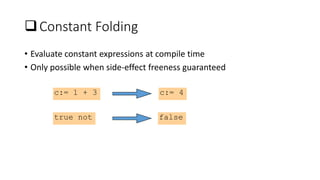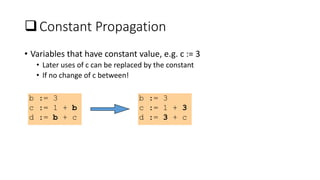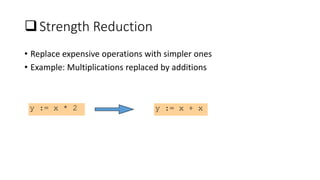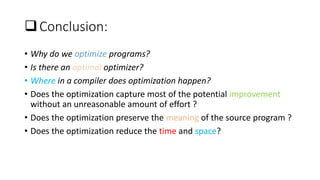1 sur 21
Publicité

### Introduction to code optimization by dipankar

1. Introduction to Code Optimization Dipankar Nalui M.SC. Computer Science Dept. of Computer Science Pondicherry University
2. Compiler • A compiler is a program that accepts a high level language and produces a target language as output (Assembly Language). Compiler Assembly Level High Level Language Language
3.  Phases of Compiler 1) Lexical Analysis 2) Syntax Analysis 3) Semantic Analysis 4) Intermediate Code Generation 5)Code Optimization 6) Code Generation
4.  Code Optimization •Optimization is the process of transforming a piece of code to make more efficient (either in terms of time or space) without changing its output or side-effects.
5. Why optimization is needed ??? • To improve intermediate code • Better target code • Executes Faster • Shorter code • Less power • Complexity : Time, Space & Cost • Efficient memory usage • Better performance.
6.  “Optimization” • The only difference visible to the code’s user should be that it runs faster and/or consumes less memory. • The name implies you are finding an "optimal“ solution— in truth, optimization aims to improve, not perfect, the result.
7. Correctness Above All! • Optimization should not change the correctness of the generated code. • Transforming the code to something that runs faster but incorrectly is of little value. • It is expected that the un-optimized and optimized variants give the same output for all inputs.
8. position = initial + rate * 60 id1 = id2 + id3 * 60 Intermediate Code T1= int-to-float(60) T2=id3*T1 T3=id2+T2 id1=T3 Eliminate int-to-float by replacing 60 by 60.0 Code Optimization T1=id3 * 60.0 Id1=id2 + t1 Code Optimizer can deduce that the conversion of 60 from int to float can be done once.
9.  The principal Sources of Optimization • Some techniques are applied to the intermediate code, to streamline, rearrange, compress, etc. • Control-Flow Analysis • Local Optimizations • Constant Folding • Constant Propagation • Operator Strength Reduction • Copy Propagation • Dead Code Elimination • Common Subexpression Elimination • Global Optimizations, Data-Flow Analysis
10. Copy Propagation Intermediate Code tmp2 = tmp1 ; tmp3 = tmp2 * tmp1; tmp4 = tmp3 ; tmp5 = tmp3 * tmp2 ; c = tmp5 + tmp4 ; The code on the left makes a copy of tmp1 in tmp2 and a copy of tmp3 in tmp4. Code Optimization tmp3 = tmp1 * tmp1 ; tmp5 = tmp3 * tmp1 ; c = tmp5 + tmp3 ; we eliminated those unnecessary copies
11. Dead Code Elimination • If an instruction’s result is never used, the instruction is considered "dead" and can be removed from the instruction stream. • tmp1 = tmp2 + tmp3 ; • if tmp1 is never used again, we can eliminate this instruction. • if tmp1 holds the result of a function call: • tmp1 = LCall _Binky; • Even if tmp1 is never used again, we cannot eliminate the instruction because we can’t be sure that called function has no side-effects.
12.  Common Sub-expression Elimination Two operations are common if they produce the same result. main() { int x, y, z; x = (1+20)* -x; y = x*x+(x/y); y = z = (x/y)/(x*x); } tmp1 = 1 + 20 ; tmp2 = -x ; x = tmp1 * tmp2 ; tmp3 = x * x ; tmp4 = x / y ; y = tmp3 + tmp4 ; tmp5 = x / y ; tmp6 = x * x ; z = tmp5 / tmp6 ; y = z ;
13. Intermediate Code tmp1 = 1 + 20 ; tmp2 = -x ; x = tmp1 * tmp2 ; tmp3 = x * x ; tmp4 = x / y ; y = tmp3 + tmp4 ; tmp5 = x / y ; tmp6 = x * x ; z = tmp5 / tmp6 ; y = z ; Code Optimization tmp2 = -x ; x = 21 * tmp2 ; tmp3 = x * x ; tmp4 = x / y ; y = tmp3 + tmp4 ; tmp5 = x / y ; z = tmp5 / tmp3 ; y = z ;
14. Algebraic Simplification • x+0 = x • 0+x = x • x*1 = x • 1*x = x • 0/x = 0 • x-0 = x • b && true = b • b && false = false • b || true = true • b || false = b
15.  Example of Algebraic Simplification b = 5 + a + 10 ; Intermediate Code _tmp0 = 5 ; _tmp1 = _tmp0 + a ; _tmp2 = _tmp1 + 10 ; b = _tmp2 ; Code Optimization _tmp0 = 15 ; _tmp1 = a + _tmp0 ; b = _tmp1 ;
16.  Constant Folding • Evaluate constant expressions at compile time • Only possible when side-effect freeness guaranteed c:= 1 + 3 c:= 4 true not false
17.  Constant Propagation • Variables that have constant value, e.g. c := 3 • Later uses of c can be replaced by the constant • If no change of c between! b := 3 c := 1 + b d := b + c b := 3 c := 1 + 3 d := 3 + c
18.  Strength Reduction • Replace expensive operations with simpler ones • Example: Multiplications replaced by additions y := x * 2 y := x + x
19.  Conclusion: • Why do we optimize programs? • Is there an optimal optimizer? • Where in a compiler does optimization happen? • Does the optimization capture most of the potential improvement without an unreasonable amount of effort ? • Does the optimization preserve the meaning of the source program ? • Does the optimization reduce the time and space?
20. Questions ??
21. Thank You …
Publicité